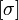# Outer automorphism group maps to automorphism group of center

Suppose$G$ is a group. Denote by$\operatorname{Out}(G)$ the outer automorphism group of$G$ and by$Z(G)$ the center of$G$. Denote by$\operatorname{Aut}(Z(G))$ the automorphism group of$Z(G)$. Then, there is a canonical homomorphism:$\operatorname{Out}(G) \to \operatorname{Aut}(Z(G))$
defined as follows: for any outer automorphism class$[\sigma]$ of$G$, pick a representative automorphism$\sigma$, and consider the restriction of$\sigma$ to$Z(G)$.
Proof that this is well defined: Since any inner automorphism restricts to the identity on$Z(G)$, the automorphism obtained by restriction is independent of the choice of representative.